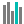#PERCENTRANK.EXC (PQL - xl)

This function returns the rank of a value in a data set as a percentage (0..1, exclusive) of the data set

• Library: PQL \ Spreadsheet \ Statistical
• Compatibility: Any content (regardless of data source) in the Tabulate spreadsheet module

#### Syntax

PERCENTRANK.EXC(array, x, OPTIONAL significance)

##### Function Arguments
 Name Description Type Optional array Array of data with numeric values that defines the relative ranking StringOrNumber x Value for which you want to find the rank in percentage Number significance Number of significant digits for the ranked percentage value; if omitted, the calculation used three significant digits Number Y